# Chapter 23 Model Basics

Author: Ron Reviewer:

## 23.2 A simple model

### 23.2.1 Exercises

1. One downside of the linear model is that it is sensitive to unusual values because the distance incorporates a squared term. Fit a linear model to the simulated data below, and visualise the results. Rerun a few times to generate different simulated datasets. What do you notice about the model?
``````sim1a <- tibble(
x = rep(1:10, each = 3),
y = x * 1.5 + 6 + rt(length(x), df = 2)
)``````

Run linear model and visualize it

``````fit <- lm(y~x, data = sim1a)
ggplot(sim1a,aes(x,y))+
geom_point(size = 2, color = "grey30")+
geom_abline(intercept = fit\$coefficients,slope = fit\$coefficients)``````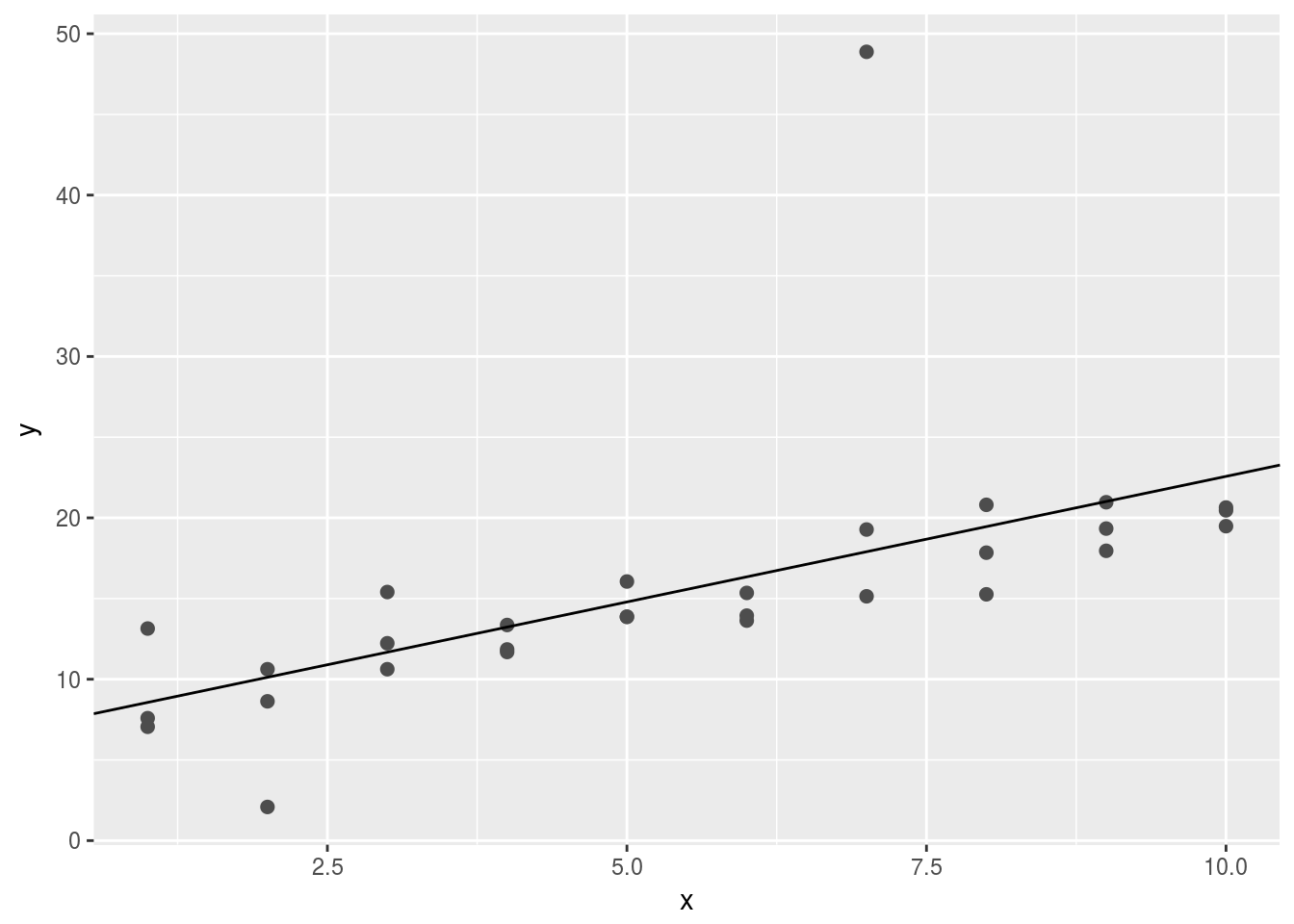Sometimes, one single abnormal value forces the fitted line deviate from the “intutively” best lines.

2.One way to make linear models more robust is to use a different distance measure. For example, instead of root-mean-squared distance, you could use mean-absolute distance:

``````model1 <- function(a, data) {
a + data\$x * a
}

measure_distance <- function(mod, data) {
diff <- data\$y - model1(mod, data)
mean(abs(diff))
}

measure_distance2 <- function(mod, data) {
diff <- data\$y - model1(mod, data)
sqrt(mean(diff ^ 2))
}``````

Compare the two measures of distance

``````sim1a <- tibble(
x = rep(1:10, each = 3),
y = x * 1.5 + 6 + rt(length(x), df = 2)
)
best1 <- optim(c(0,0), measure_distance, data = sim1a)
best2 <- optim(c(0,0), measure_distance2, data = sim1a)

ggplot(sim1a, aes(x, y)) +
geom_point(size = 2, colour = "grey30") +
geom_abline(intercept = best1\$par, slope = best1\$par, color = "red")+
geom_abline(intercept = best2\$par,slope = best2\$par, color = "cyan")``````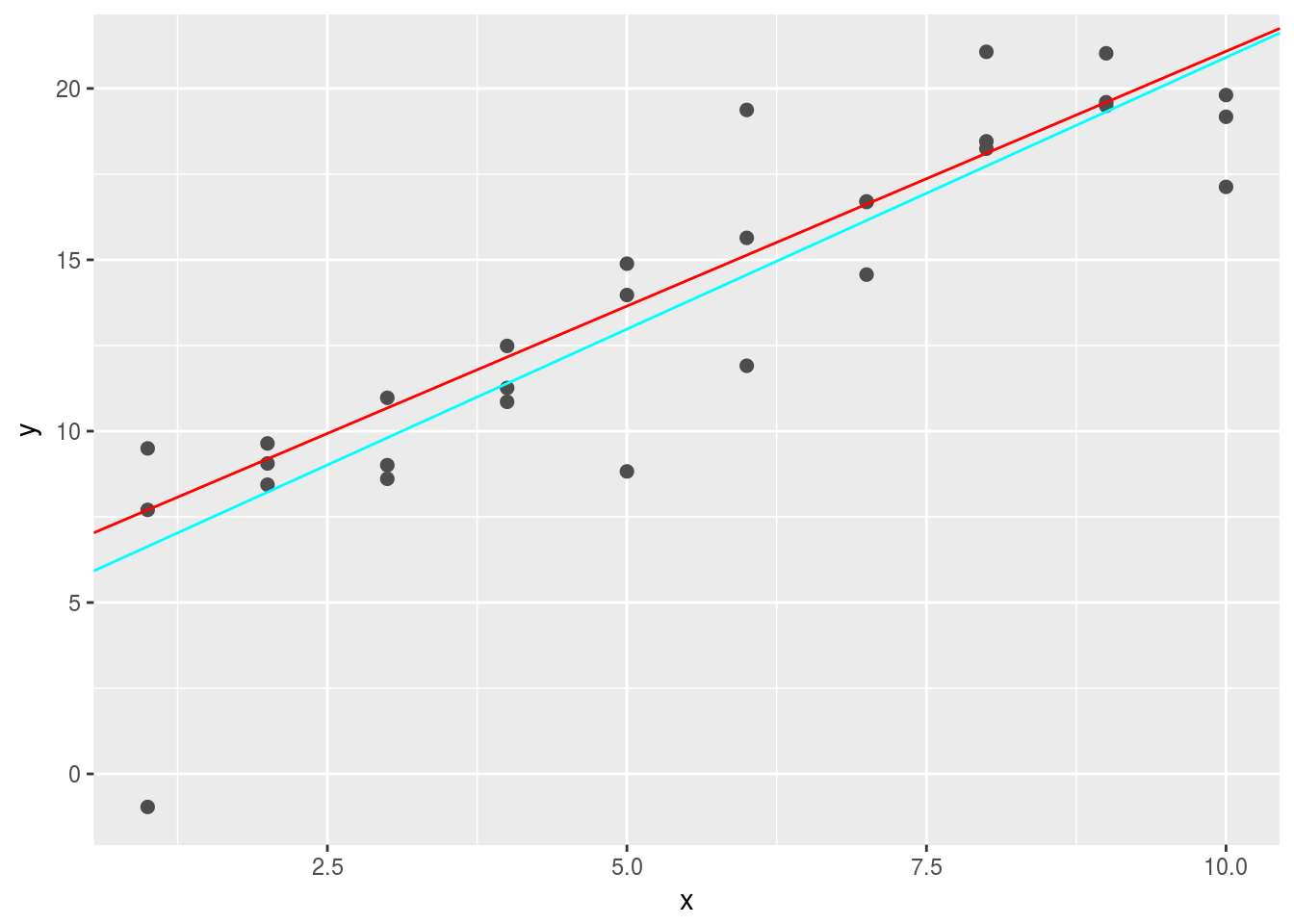When there are many abnormal points, the `cyan` line will perform better using absolute distances. It is better because measn-square-distance tends to overemphasize abnormal values. 3. One challenge with performing numerical optimisation is that it’s only guaranteed to find one local optima. What’s the problem with optimising a three parameter model like this?

``````model1 <- function(a, data) {
a + data\$x * a + a
}

measure_distance3 <- function(mod, data) {
diff <- data\$y - model1(mod, data)
sqrt(mean(diff ^ 2))
}

sim1a <- tibble(
x = rep(1:10, each = 3),
y = x * 1.5 + 6 + rt(length(x), df = 2)
)
best3 <- optim(c(0,0,0), measure_distance3, data = sim1a)
best3\$par``````
``##  -3.706809  1.475589  9.937808``
``````ggplot(sim1a, aes(x, y)) +
geom_point(size = 2, colour = "grey30") +
geom_abline(intercept = best3\$par + best3\$par, slope = best3\$par, color = "red")``````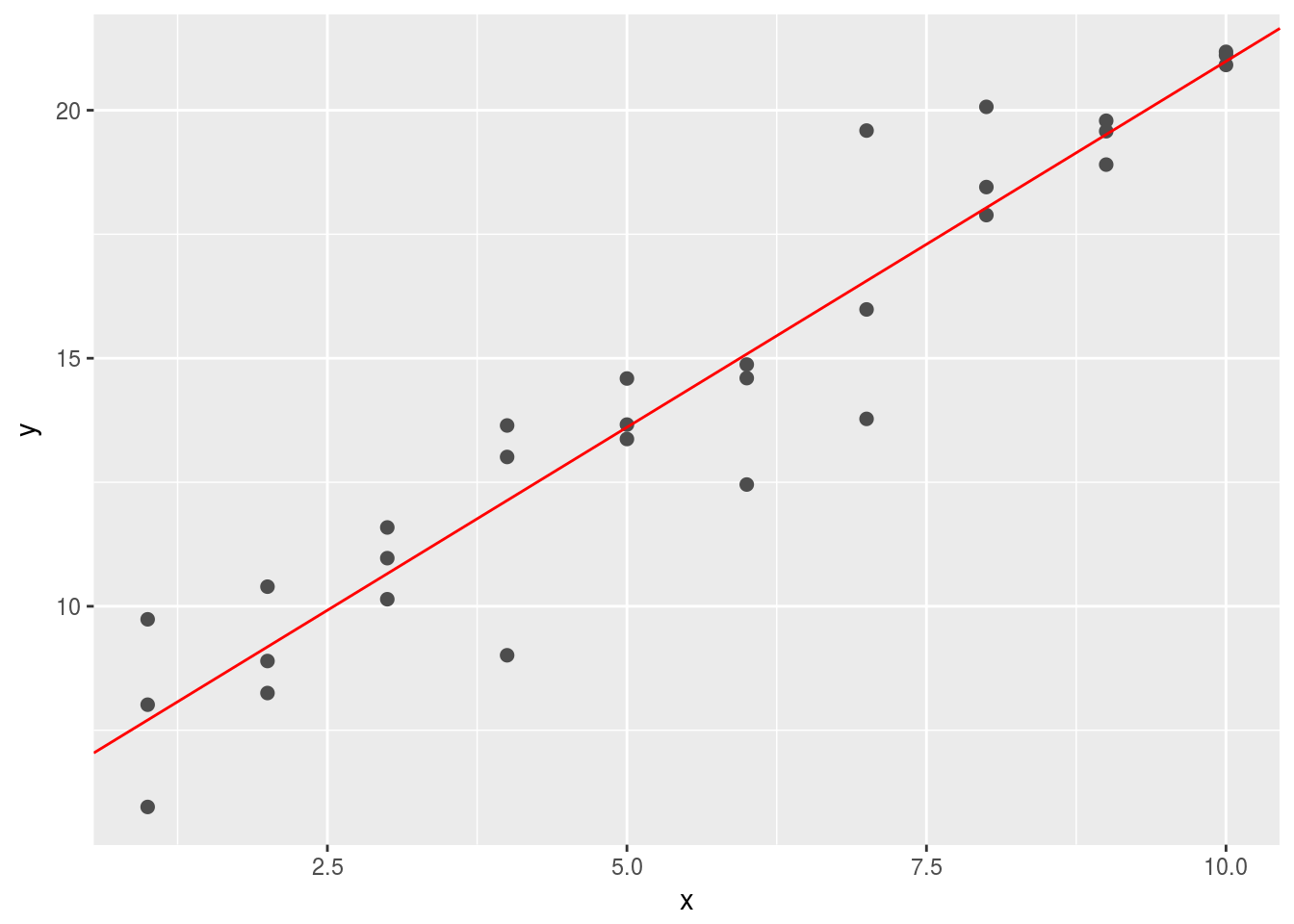There are essentially infinitely many solutions since the model we built contains redudant information in `a` and `a`.

## 23.3 Visualising models

### 23.3.3 Exercises

1.Instead of using `lm()` to fit a straight line, you can use `loess()` to fit a smooth curve. Repeat the process of model fitting, grid generation, predictions, and visualisation on sim1 using `loess()` instead of `lm()`. How does the result compare to `geom_smooth()`?

``````fit1 <- lm(y~x, data = sim1)
fit2 <- loess(y~x, data = sim1,degree = 2)

grid <- sim1 %>% data_grid(x)
grid1 <- grid %>%

grid2 <- grid %>%

plot the predictions

``````ggplot(sim1,aes(x=x))+
geom_point(aes(y=y))+
geom_line(data = grid1, aes(y = pred), color = 'red')+
geom_smooth(data = grid2, aes(y = pred),color = 'cyan')``````
``## `geom_smooth()` using method = 'loess'``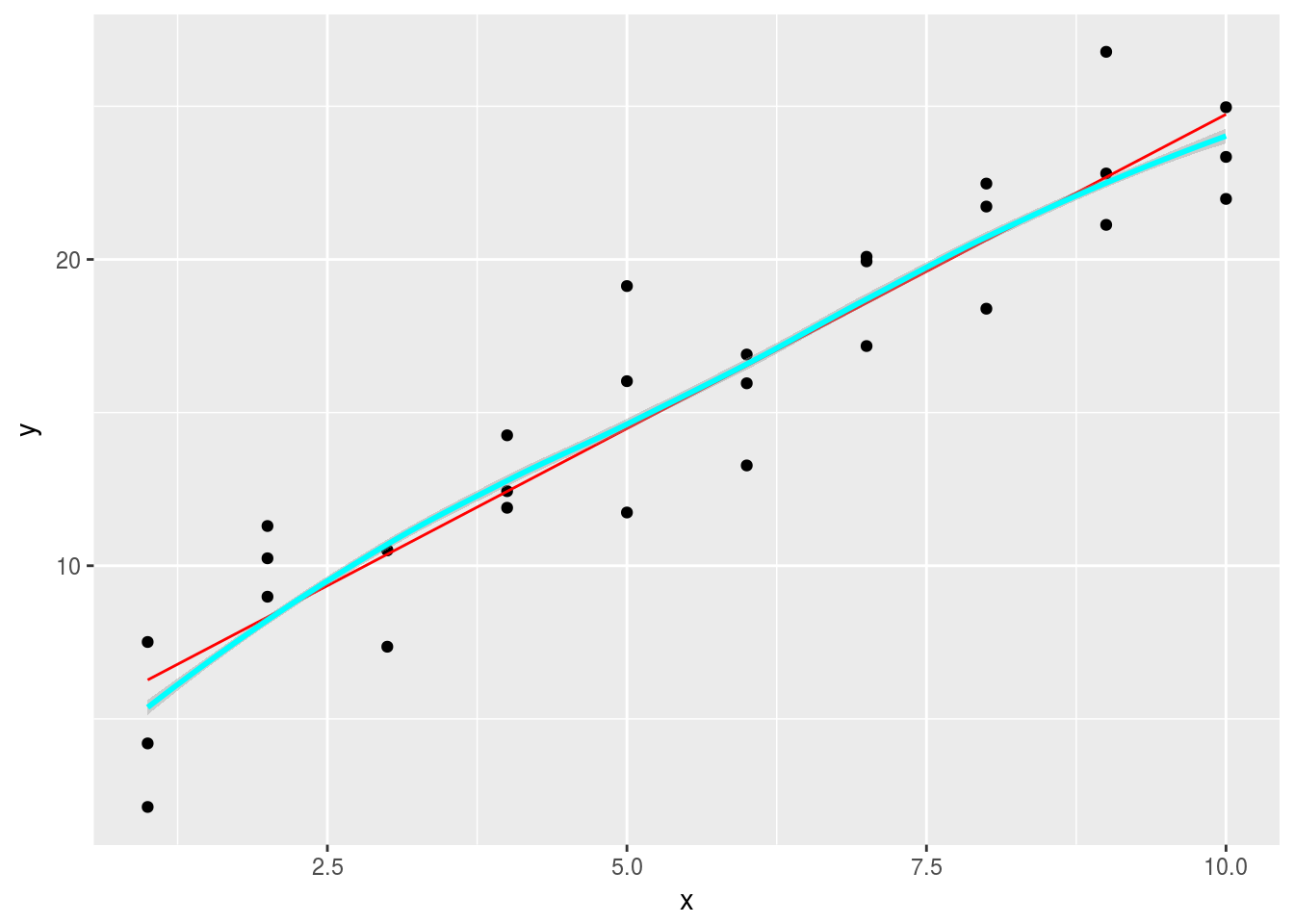Plot the residuals

``````ggplot() +
geom_freqpoly(data = sim1_1, aes(resid),binwidth = 0.5,color = 'red') +
geom_freqpoly(data = sim1_2, aes(resid),binwidth = 0.5, color = 'cyan')``````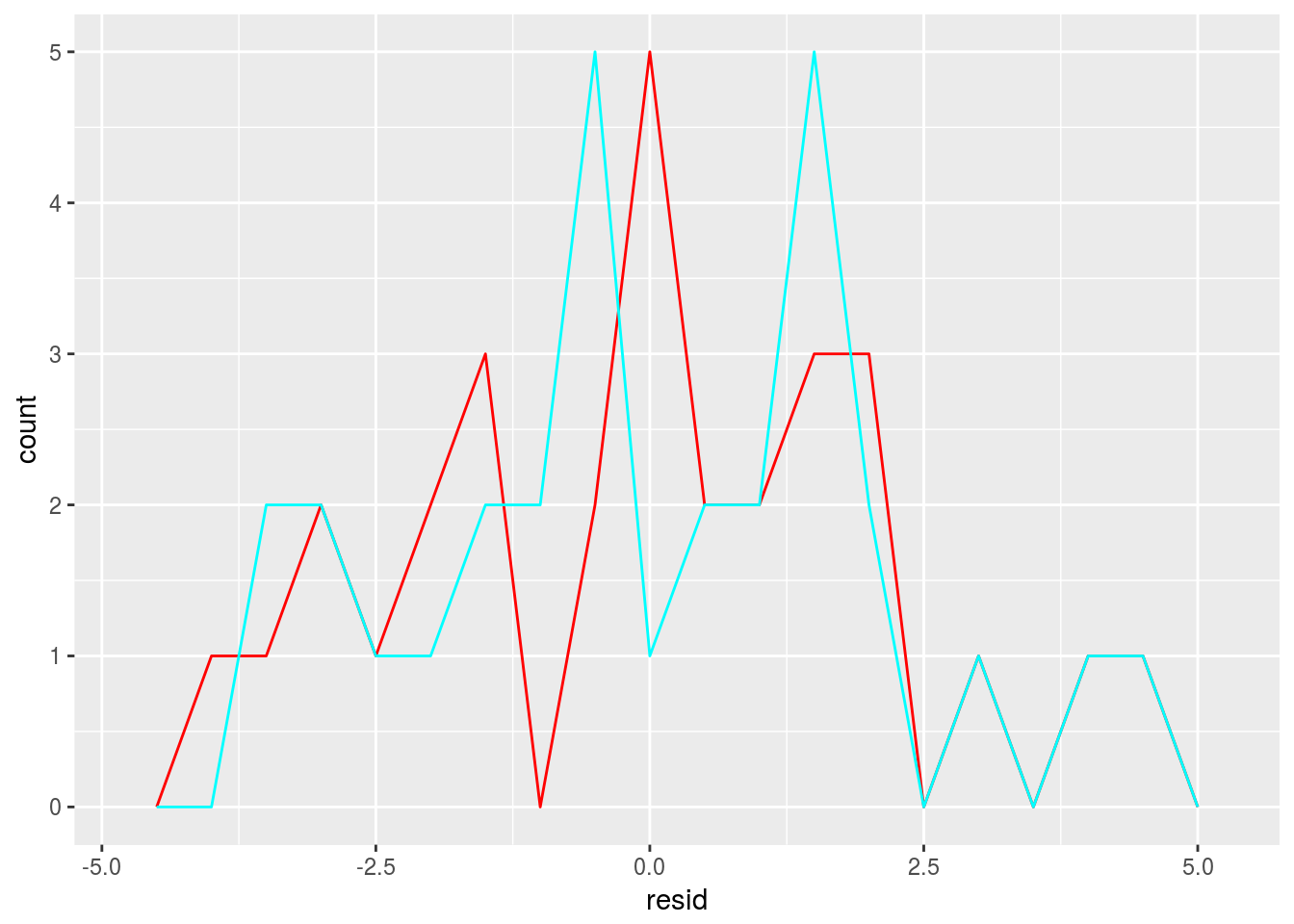2. `add_predictions()` is paired with `gather_predictions()` and `spread_predictions()`. How do these three functions differ?

Similar to the idea of `gather` and `spread` in the `tidyr` package. `spread_predicitions` will create a fat table with each model creating a column of its own prediction. `gather_predictions` will create two columns with one columns indicating the type of the model and another one it prediction, resulting in a tall table.

1. What does `geom_ref_line()` do? What package does it come from? Why is displaying a reference line in plots showing residuals useful and important?

`geom_ref_line()` add a reference line in the graph, it comes from `modelr` package. It is useful for you to detect the trend and disribution of residuals visually.

1. Why might you want to look at a frequency polygon of absolute residuals? What are the pros and cons compared to looking at the raw residuals?
``````ggplot() +
geom_freqpoly(data = sim1_1, aes(abs(resid)),binwidth = 0.5,color = 'red')``````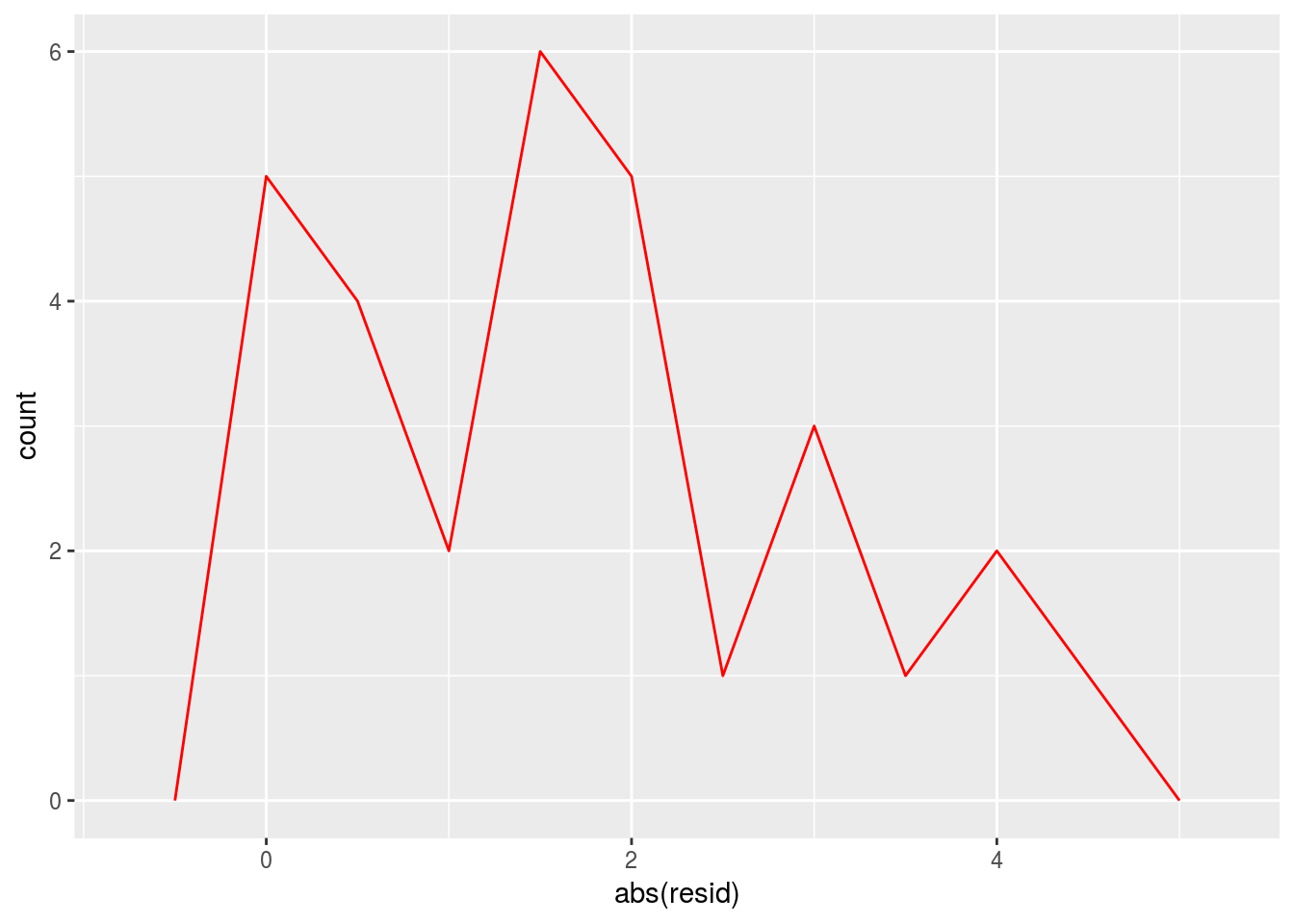You want to check the absolute residuals because it helps to see the overall quality of the prediction but it won’t give you the hint about the distribution of residuals with respect to 0. For example, it may be possible that there is only one large possitve residual and many small negative ones.

## 23.4 Formulas and model families

### 23.4.5 Exercises

1.What happens if you repeat the analysis of `sim2` using a model without an intercept. What happens to the model equation? What happens to the predictions?

``````mod1 <- lm(y~x - 1, data = sim2)
mod2 <- lm(y~x, data = sim2)
mod1\$coefficients``````
``````##       xa       xb       xc       xd
## 1.152166 8.116039 6.127191 1.910981``````
``mod2\$coefficients``
``````## (Intercept)          xb          xc          xd
##   1.1521664   6.9638728   4.9750241   0.7588142``````
``````grid1 <- sim2 %>%
data_grid(x)%>%
gather_predictions(mod1,mod2)
grid1``````
``````## # A tibble: 8 x 3
##   model     x     pred
##   <chr> <chr>    <dbl>
## 1  mod1     a 1.152166
## 2  mod1     b 8.116039
## 3  mod1     c 6.127191
## 4  mod1     d 1.910981
## 5  mod2     a 1.152166
## 6  mod2     b 8.116039
## 7  mod2     c 6.127191
## 8  mod2     d 1.910981``````

The equation will have no intercept term.

``````sim2 %>%
ggplot(aes(x))+
geom_point(aes(y=y))+
geom_point(data = grid1, aes(y = pred),color = "red",size = 4)+
facet_grid(~model)``````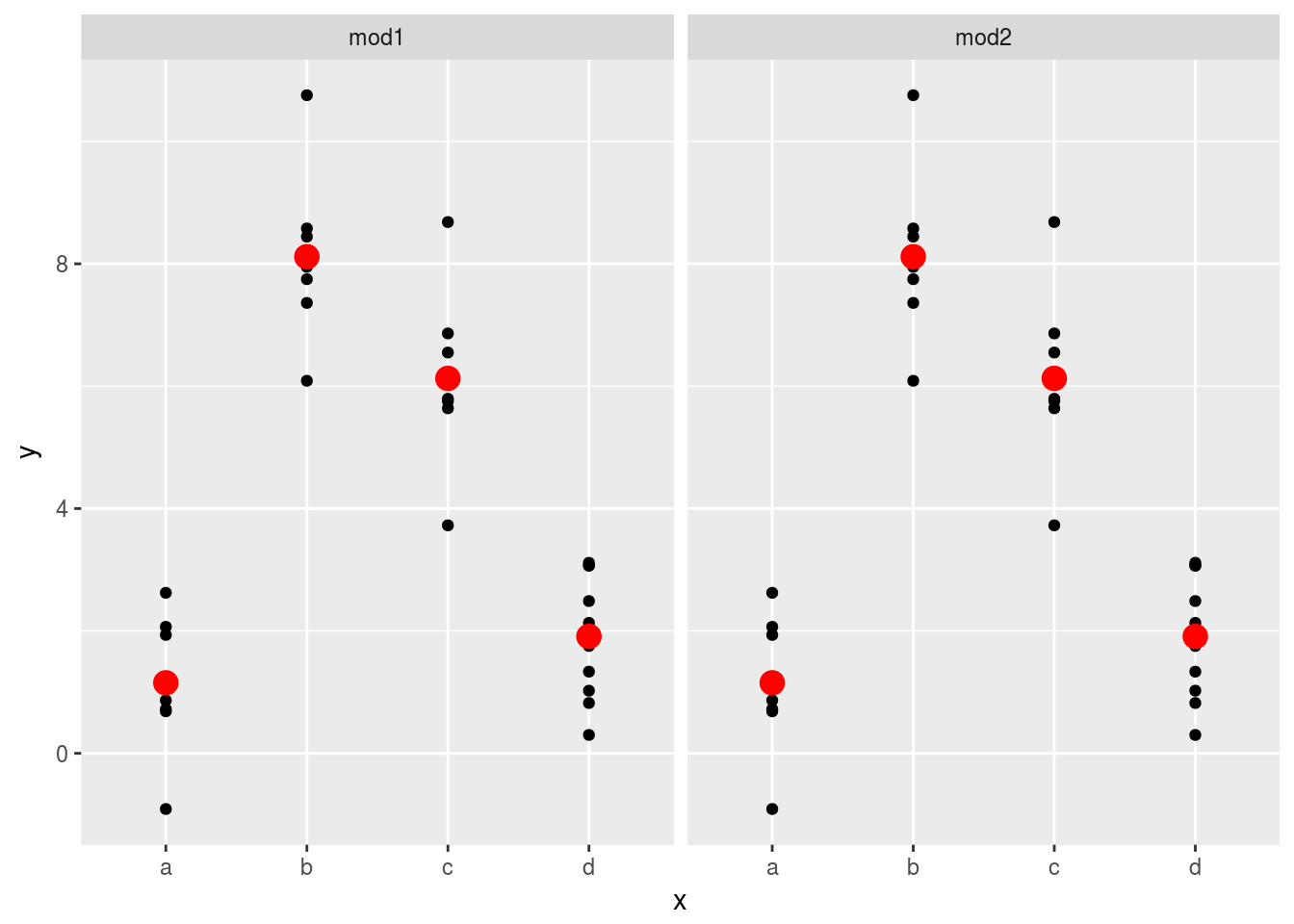However, the prediction doesn’t change. Categorical predictors are not affected by the removal of intercept terms.

2.Use `model_matrix()` to explore the equations generated for the models I fit to `sim3` and `sim4`. Why is `*` a good shorthand for interaction?

``````# sim3

model_matrix(data = sim3, y ~ x1 + x2)``````
``````## # A tibble: 120 x 5
##    `(Intercept)`    x1   x2b   x2c   x2d
##            <dbl> <dbl> <dbl> <dbl> <dbl>
##  1             1     1     0     0     0
##  2             1     1     0     0     0
##  3             1     1     0     0     0
##  4             1     1     1     0     0
##  5             1     1     1     0     0
##  6             1     1     1     0     0
##  7             1     1     0     1     0
##  8             1     1     0     1     0
##  9             1     1     0     1     0
## 10             1     1     0     0     1
## # ... with 110 more rows``````
``model_matrix(data = sim3, y ~ x1 * x2)``
``````## # A tibble: 120 x 8
##    `(Intercept)`    x1   x2b   x2c   x2d `x1:x2b` `x1:x2c` `x1:x2d`
##            <dbl> <dbl> <dbl> <dbl> <dbl>    <dbl>    <dbl>    <dbl>
##  1             1     1     0     0     0        0        0        0
##  2             1     1     0     0     0        0        0        0
##  3             1     1     0     0     0        0        0        0
##  4             1     1     1     0     0        1        0        0
##  5             1     1     1     0     0        1        0        0
##  6             1     1     1     0     0        1        0        0
##  7             1     1     0     1     0        0        1        0
##  8             1     1     0     1     0        0        1        0
##  9             1     1     0     1     0        0        1        0
## 10             1     1     0     0     1        0        0        1
## # ... with 110 more rows``````
``````# sim4
model_matrix(data = sim4, y ~ x1 + x2)``````
``````## # A tibble: 300 x 3
##    `(Intercept)`    x1         x2
##            <dbl> <dbl>      <dbl>
##  1             1    -1 -1.0000000
##  2             1    -1 -1.0000000
##  3             1    -1 -1.0000000
##  4             1    -1 -0.7777778
##  5             1    -1 -0.7777778
##  6             1    -1 -0.7777778
##  7             1    -1 -0.5555556
##  8             1    -1 -0.5555556
##  9             1    -1 -0.5555556
## 10             1    -1 -0.3333333
## # ... with 290 more rows``````
``````#model_matrix(data = sim4, y ~ x1 + x2 + I(x1*x2))
model_matrix(data = sim4, y ~ x1 * x2)``````
``````## # A tibble: 300 x 4
##    `(Intercept)`    x1         x2   `x1:x2`
##            <dbl> <dbl>      <dbl>     <dbl>
##  1             1    -1 -1.0000000 1.0000000
##  2             1    -1 -1.0000000 1.0000000
##  3             1    -1 -1.0000000 1.0000000
##  4             1    -1 -0.7777778 0.7777778
##  5             1    -1 -0.7777778 0.7777778
##  6             1    -1 -0.7777778 0.7777778
##  7             1    -1 -0.5555556 0.5555556
##  8             1    -1 -0.5555556 0.5555556
##  9             1    -1 -0.5555556 0.5555556
## 10             1    -1 -0.3333333 0.3333333
## # ... with 290 more rows``````

`*` is good because 1. It is simple and efficient to treat categorical predictors, which is tedious to do using `+`. Or even impossible? 2. It is simple to create interaction term for continuous varaibles too.

1. Using the basic principles, convert the formulas in the following two models into functions. (Hint: start by converting the categorical variable into 0-1 variables.)
``````mod1 <- lm(y ~ x1 + x2, data = sim3)
mod2 <- lm(y ~ x1 * x2, data = sim3)``````

Convert `x2` to bianry variables. Thanks to this StackOverflow answer

``````library(tidyr)
sim3 <- sim3 %>%
mutate(present = 1)%>%
``````mod1 <- lm(y ~ x1 + a + b + c + d, data = sim3)
mod2 <- lm(y ~ x1*a*b*c*d, data = sim3)
# all possible combinations
head(model.matrix(data = sim3, y ~ x1*a*b*c*d))``````
``````##   (Intercept) x1 a b c d x1:a x1:b a:b x1:c a:c b:c x1:d a:d b:d c:d
## 1           1  1 1 0 0 0    1    0   0    0   0   0    0   0   0   0
## 2           1  1 0 0 0 1    0    0   0    0   0   0    1   0   0   0
## 3           1  1 0 0 1 0    0    0   0    1   0   0    0   0   0   0
## 4           1  1 0 1 0 0    0    1   0    0   0   0    0   0   0   0
## 5           1  1 1 0 0 0    1    0   0    0   0   0    0   0   0   0
## 6           1  1 0 0 0 1    0    0   0    0   0   0    1   0   0   0
##   x1:a:b x1:a:c x1:b:c a:b:c x1:a:d x1:b:d a:b:d x1:c:d a:c:d b:c:d
## 1      0      0      0     0      0      0     0      0     0     0
## 2      0      0      0     0      0      0     0      0     0     0
## 3      0      0      0     0      0      0     0      0     0     0
## 4      0      0      0     0      0      0     0      0     0     0
## 5      0      0      0     0      0      0     0      0     0     0
## 6      0      0      0     0      0      0     0      0     0     0
##   x1:a:b:c x1:a:b:d x1:a:c:d x1:b:c:d a:b:c:d x1:a:b:c:d
## 1        0        0        0        0       0          0
## 2        0        0        0        0       0          0
## 3        0        0        0        0       0          0
## 4        0        0        0        0       0          0
## 5        0        0        0        0       0          0
## 6        0        0        0        0       0          0``````
1. For `sim4`, which of `mod1` and `mod2` is better? I think `mod2` does a slightly better job at removing patterns, but it’s pretty subtle. Can you come up with a plot to support my claim?

Let’s check the distribution of the residuals.

``````mod1 <- lm(y ~ x1 + x2, data = sim4)
mod2 <- lm(y ~ x1 * x2, data = sim4)

grid <- sim4 %>%
data_grid(x1,x2)
grid <- grid %>%
gather_predictions(mod1, mod2)

sim4 <- sim4 %>% gather_residuals(mod1,mod2)``````

Plot the residual’s distribution

``````med1 <- median((sim4 %>% filter(model == "mod1"))\$resid)
med2 <- median((sim4 %>% filter(model == "mod2"))\$resid)
ggplot(sim4, aes(resid,color = model))+
geom_histogram(binwidth = 0.01,position = "stack")``````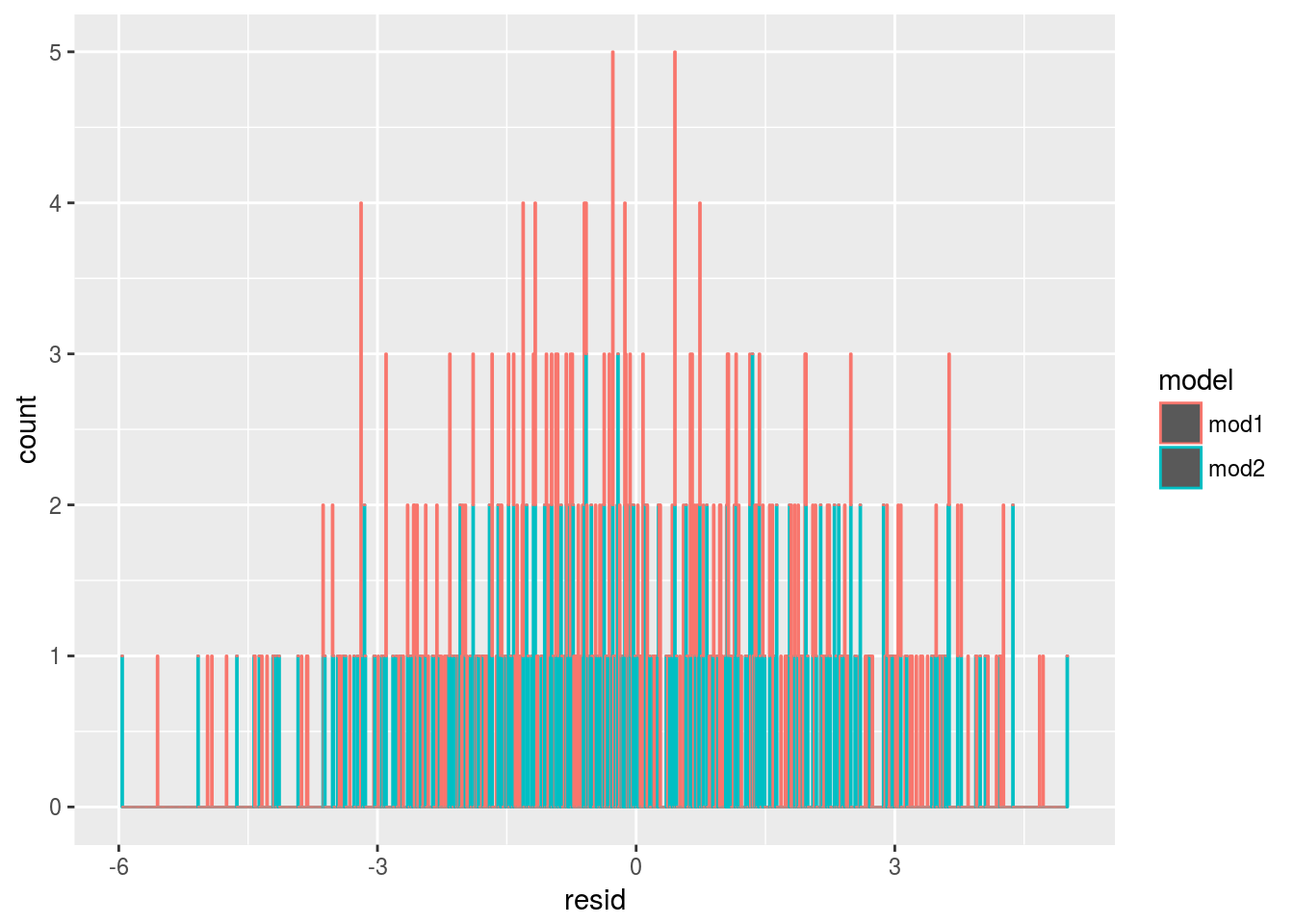From the exploratory analysis, we may plot the residue as a function of `x1`

``````sim4 %>% group_by(model)%>%
summarize(mean_abs_resid = mean(abs(resid)))``````
``````## # A tibble: 2 x 2
##   model mean_abs_resid
##   <chr>          <dbl>
## 1  mod1       1.708070
## 2  mod2       1.670054``````
``````# the mean of absolute residual for mod2 is smaller for mod1
sim4 %>%
ggplot(aes(y=abs(resid),x = as.factor(x1)))+
geom_boxplot()+
facet_grid(~model)``````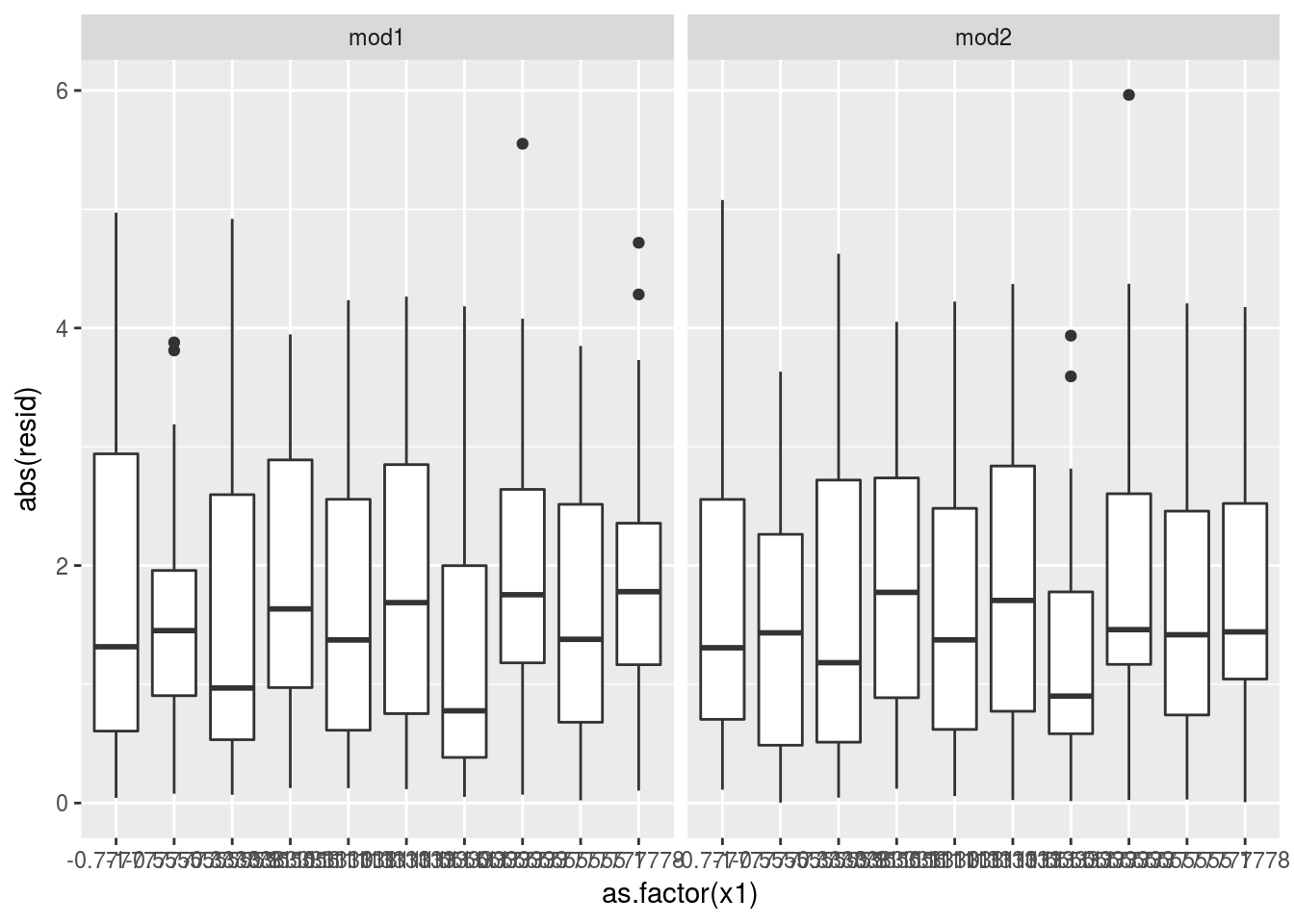It is not that obvious but visually detectable.

## 23.5 Missing values

Notes on handling NA behaviors.

``````# get warning
options(na.action = na.warn)
# suppress warning in an operation
lm(y~x,data = sim1, na.action = na.exclude)``````
``````##
## Call:
## lm(formula = y ~ x, data = sim1, na.action = na.exclude)
##
## Coefficients:
## (Intercept)            x
##       4.221        2.052``````

## 23.6 Other model families

Note:

1. Generalised linear models
1. remove assumptions that response is continuous and error is normally distributed.
2. define distance metric based on the statistical idea of likelihood.
1. incorporate arbitrary smooth functions beyond `GLM`.